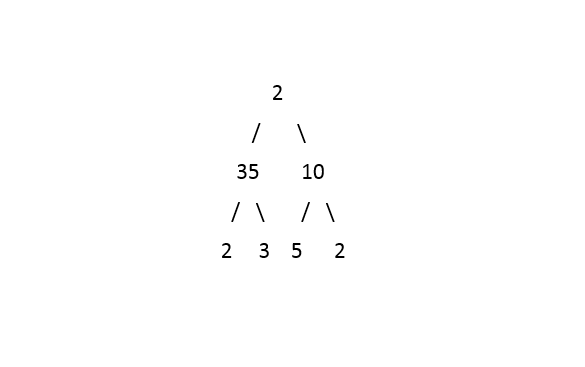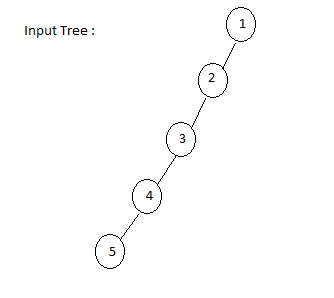6
Avg. time to solve
35 min
Success Rate
65%

Problem Statement

The right view of a Binary Tree is a set of nodes visible when the tree is viewed from the Right side and the nodes are printed from top to bottom order.

Input Format:
The first line contains an integer 'T' which denotes the number of test cases or queries to be run. Then the test cases follow.

The only line of input contains the elements of the tree in the level order form separated by a single space.

If any node does not have left or right child, take -1 in its place. Refer to the example below.

Example:

Elements are in the level order form. The input consists of values of nodes separated by a single space in a single line. In case a node is null, we take -1 on its place.

For example, the input for the tree depicted in the below image would be :1
2 3
4 -1 5 6
-1 7 -1 -1 -1 -1
-1 -1

Explanation :
Level 1 :
The root node of the tree is 1

Level 2 :
Left child of 1 = 2
Right child of 1 = 3

Level 3 :
Left child of 2 = 4
Right child of 2 = null (-1)
Left child of 3 = 5
Right child of 3 = 6

Level 4 :
Left child of 4 = null (-1)
Right child of 4 = 7
Left child of 5 = null (-1)
Right child of 5 = null (-1)
Left child of 6 = null (-1)
Right child of 6 = null (-1)

Level 5 :
Left child of 7 = null (-1)
Right child of 7 = null (-1)

The first not-null node (of the previous level) is treated as the parent of the first two nodes of the current level. The second not-null node (of the previous level) is treated as the parent node for the next two nodes of the current level and so on.

The input ends when all nodes at the last level are null (-1).
Note :
The above format was just to provide clarity on how the input is formed for a given tree.

The sequence will be put together in a single line separated by a single space. Hence, for the above-depicted tree, the input will be given as:

1 2 3 4 -1 5 6 -1 7 -1 -1 -1 -1 -1 -1
Output Format :
For each test case,  print the right view of the tree.

Output for every test case will be in a separate line.

Note:

You do not need to print anything, it has already been taken care of. Just implement the given function.
Constraints:
1 <= T <= 100
1 <= N <= 10^5
-10^9 <= data <= 10^9

Where 'N' is the number of nodes in the tree and 'data' is the value of a node in the given tree.

Time Limit: 1 sec
Sample Input 1 :
1
2 35 10 2 3 5 2 -1 -1 -1 -1 -1 -1 -1 -1
2 10 2
Explanation of The Sample Input 1:The right view of the tree contains all the extreme-right elements in each level of the tree, including the head of the tree.
Sample Input 2 :
1
1 2 -1 3 -1 4 -1 5 -1 -1 -1
1 2 3 4 5
Explanation of The Sample Input 2 :Console